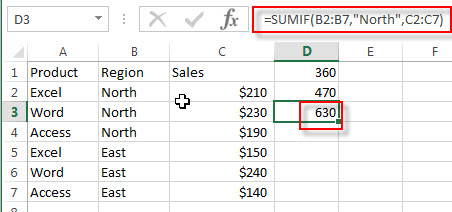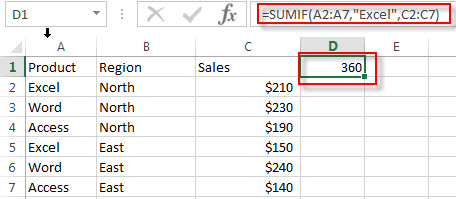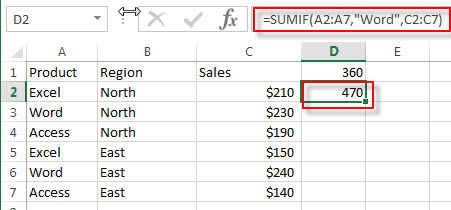# Excel SUMIF Function

This post will guide you how to use Excel SUMIF function with syntax and examples in Microsoft excel.

### Description

The Excel SUMIF function sum the numbers in the range of cells that meet a single criteria that you specify. You can use the SUMIF function to sum cells that based on the dates, numbers or texts based on one criteria in Excel.

The SUMIF function will extend the capabilities of the SUM function by giving you a choice that you want only sum those values that meet a single criteria.

The SUMIF function is a build-in function in Microsoft Excel and it is categorized as a Math and Trigonometry Function.

The SUMIF function is available in Excel 2016, Excel 2013, Excel 2010, Excel 2007, Excel 2011 for Mac.

### Syntax

The syntax of the SUMIF function is as below:

`=SUMIF (range, criteria, [sum_range])`

Where the SUMIF function arguments are:

• Range -This is a required argument. The range of cells that you want to apply the criteria against. And the cells in each range must be numbers, or names, arrays, or references that contain numbers. And if you provide the blank and text values for range argument, the values will be ignored by the SUMIF function.
• Criteria -This is a required argument. The criteria used to determine which cells in the range to be summed.
• sum_range – This is an optional argument. The actual cells to sum together, and if this argument is omitted, and the SUMIF function will use the range as the sum_range, it means that the cells in range are added together.

Note:

• Any criteria that includes logical or math symbols must be enclosed in double quotation marks.
• The numeric criteria can be supplied without quotation marks.
• You can use the wildcard characters in the criteria argument.

### Excel SUMIF Function Examples

The below examples will show you how to use Excel SUMIF Function to sum the values in a range of cells based on one criteria.

Suppose that you have a sales table as shown below:

 Product Region Sales Excel North \$210 Word North \$230 Access North \$190 Excel East \$150 Word East \$240 Access East \$140

1# to sum of the sales of the product “Excel” in all regions, enter the following formula in Cell D1.

`=SUMIF(A2:A7,"Excel",C2:C7)`2# to sum of the sales of the product “Word” in all regions, enter the following formula in Cell D2.

`=SUMIF(A2:A7,"Word",C2:C7)`3# to get total sales from North region, enter the following formula in Cell D3.

`=SUMIF(B2:B7,"North",C2:c7)`### Related Functions

• Excel SUM Function
The Excel SUM function will adds all numbers in a range of cells and returns the sum of these values. You can add individual values, cell references or ranges in excel.The syntax of the SUM function is as below:= SUM(number1,[number2],…)…
• Excel SUMIFS Function
The Excel SUMIFS function sum the numbers in the range of cells that meet a single or multiple criteria that you specify. The syntax of the SUMIFS function is as below:=SUMIFS (sum_range, criteria_range1, criteria1, [criteria_range2, criteria2], …)…
• Excel SUMSQ Function
The Excel SUMSQ function returns the sum of squares of a given set of arguments.The syntax of the SUMSQ function is as below:=SUMSQ(number1, [number2], …)…
• Excel SUMX2MY2 Function
The Excel SUMX2MY2 function returns the sum of the difference of squares between two supplied arrays.The syntax of the SUMX2MY2 function is as below:=SUMX2MY2 (array_x, array_y)…

### Related Examples

• Sum Values by Group
Assuming that you have a table that contain the product name and its sales result. And you have group by those values based on the product name, then you want to sum values based one each product name. You can create a new Excel formula based on the IF function and SUMIF function.…
• SUMIF When Partial Text String Match
If you want to sum cell values in column B and that match one criteria that the cell in the same row contains the partial text string “exc” in column A. You need to create a new formula based on the SUMIF function to achieve the result. .…
• Sumif with 3D Reference for Multiple Worksheet
If you want to sum 3d references across the different worksheet, and you need to create a complex formula based on the SUMPRODUCT function, the SUMIF function, and the INDIRECT function..….
• Sum if Cells Begin with Specific Text or Number in Another Cells
Assuming that you have a list of data A1:B4, and you want to sum all cells in column B if cells in column A begin with a specific text “excel” or a specific number “201”.You also can create a formula based on the SUMPRODUCT function and the LEFT functio..…
• Sum Values in a Column based on Criteria in Another Column
To use a formula to sum values in Column B based on Column A, you can create a formula based on the SUMIF function.You can also create an Excel Pivot Table to sum values based on another column……
• Sum Values between Two Dates
If you want to quickly sum values between two dates in Excel, you can create a formula based on the SUMIF function……
• Sum Multiple Columns based on One Criteria
You need to create a formula based on the SUM function to sum sale values for all two months in a newly helper column. And then create another formula based on the SUMIF function to sum total values for product “excel”…
• Sum the Absolute Values
Assuming that you have a list of data in range B1:B6 that contain negative numbers and positive numbers, and you want to sum all absolute values in these range of cells, You can use a formula based on the SUMIF function to achieve the result….